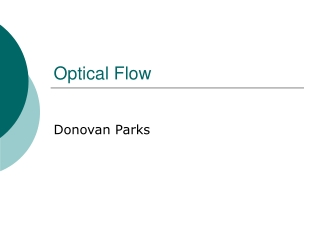DownloadDownload PresentationOptical Flow

# Optical Flow

Download Presentation## Optical Flow

- - - - - - - - - - - - - - - - - - - - - - - - - - - E N D - - - - - - - - - - - - - - - - - - - - - - - - - - -
##### Presentation Transcript

1. Optical Flow Donovan Parks

2. What is optical flow? • a method for estimating the motion of objects within an image sequence • answers the question “how are my pixels (objects) moving?”

3. Where is optical flow used? • widely used in computer vision: • motion detection • camera jitter correction • video compression • video segmentation • basis on many special effects used in the movie industry

4. Why is calculating optical flow difficult?The Aperture Problem • often insufficient information in a local area to fully determine motion

5. Apparent vs. real motion

6. How is optical flow calculated?

7. Motion constraint equation • Ix, Iy, and It are just intensity derivatives • easily calculated for images • H&S suggest: • vx, vy are the pixel velocities we want to find Problem One equation with two unknowns!

8. What constraint do Horn and Schunck suggest? • Global smoothness constraint on velocity field • Why? • Neighbouring pixels from a rigid object must have similar velocities • Therefore, the velocity field in the image should vary smoothly in most places

9. Horn-Schunck cont… • λ controls the influence of the smoothness constraint • Iterative solution exists to find the minimum • all the ugly details are in the paper!

10. Example: Optical Flow in Practice

11. Example: Sparse Optical Flow Example by David M. Stavens (http://ai.stanford.edu/~dstavens/)

12. Discussion: A word of caution • Brightness constancy assumption requires: • constant illumination conditions • objects to be at least locally rigid • Higher level processing often needed to “weed out” usable information from an optical flow algorithm

13. Conclusion • Major contribution of H&S: first to propose formal method for calculating optical flow • Critical point in calculating optical flow is finding another “good” equation to go along with the motion constraint equation

14. Questions? Thank you!